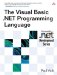# VariantType Enumeration

 <  Day Day Up  >

 The VariantType enumeration is used by the VarType function in the Information module to indicate the equivalent COM Variant type of a value. ` Empty = 0 ` Equivalent to the Variant type Empty. Constant equivalent: vbEmpty . ` Null = 1 ` Equivalent to the Variant type Null. Constant equivalent: vbNull . ` Short = 2 ` Equivalent to the Variant type Short. Constant equivalent: vbShort . ` Integer = 3 ` Equivalent to the Variant type Integer. Constant equivalent: vbInteger . ` Single = 4 ` Equivalent to the Variant type Single. Constant equivalent: vbSingle . ` Double = 5 ` Equivalent to the Variant type Double. Constant equivalent: vbDouble . ` Currency = 6 ` Equivalent to the Variant type Currency. Constant equivalent: vbCurrency . ` Date = 7 ` Equivalent to the Variant type Date. Constant equivalent: vbDate . ` String = 8 ` Equivalent to the Variant type String. Constant equivalent: vbString . ` Object = 9 ` Equivalent to the Variant type Object. Constant equivalent: vbObject . ` Error = 10 ` Equivalent to the Variant type Error. Constant equivalent: vbError . ` Boolean = 11 ` Equivalent to the Variant type Boolean. Constant equivalent: vbBoolean . ` Variant = 12 ` Equivalent to the Variant type Variant. Constant equivalent: vbVariant . ` DataObject = 13 ` Equivalent to the Variant type DataObject. Constant equivalent: vbDataObject . ` Decimal = 14 ` Equivalent to the Variant type Decimal. Constant equivalent: vbDecimal . ` Byte = 17 ` Equivalent to the Variant type Byte. Constant equivalent: vbByte . ` Char = 18 ` Equivalent to the Variant type Char. Constant equivalent: vbChar . ` Long = 20 ` Equivalent to the Variant type Long. Constant equivalent: vbLong . ` UserDefinedType = 36 ` Equivalent to the Variant type UserDefinedType. Constant equivalent: vbUserDefinedType . ` Array = 8192 ` Equivalent to a SafeArray of the specified type. Constant equivalent: vbArray .
 <  Day Day Up  >The Visual Basic .NET Programming Language
ISBN: 0321169514
EAN: 2147483647
Year: 2004
Pages: 173
Authors: Paul Vick

Similar book on Amazon